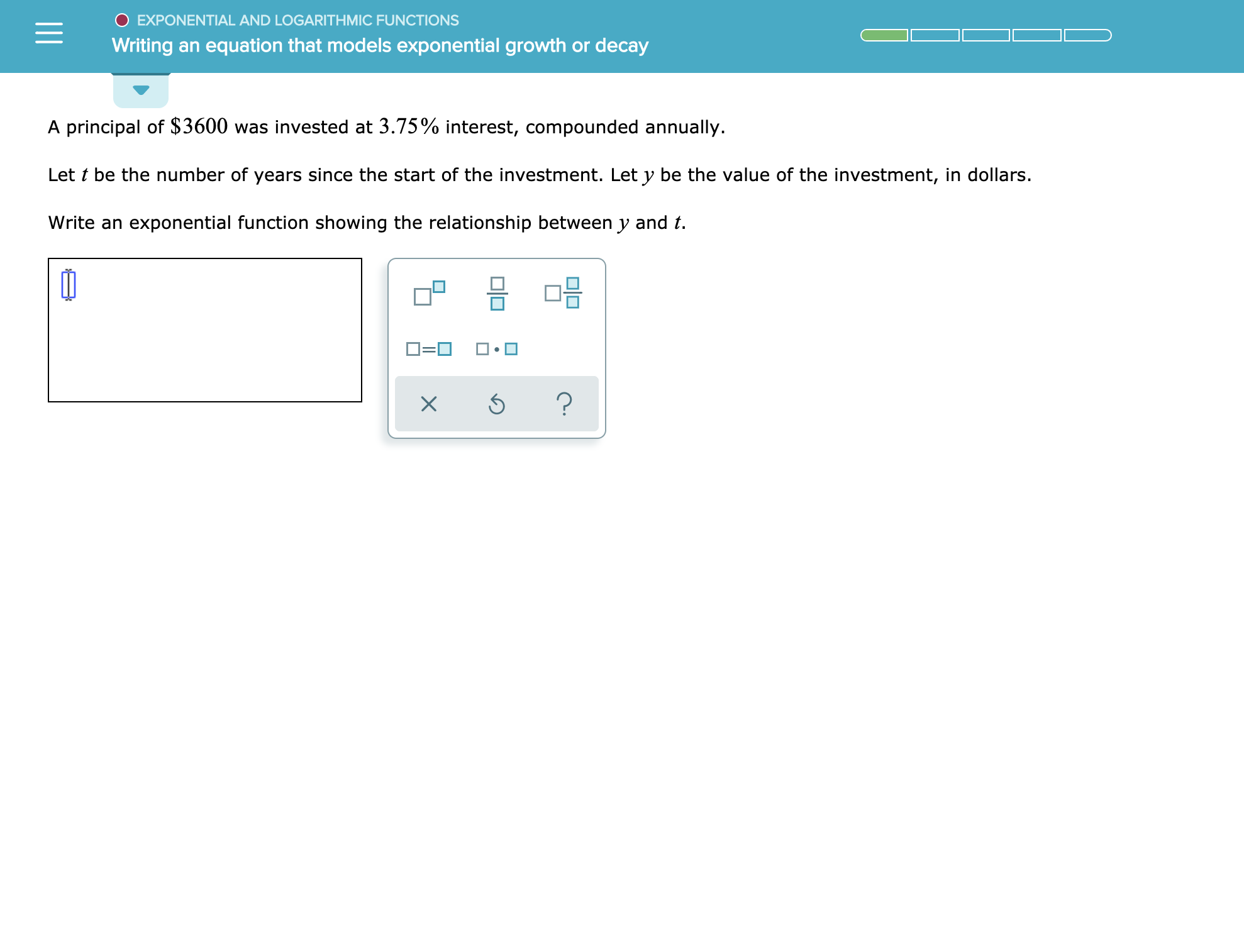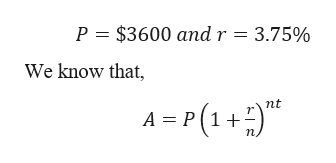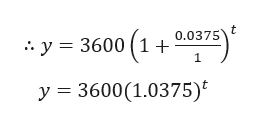EXPONENTIAL AND LOGARITH MIC FUNCTIONSWriting an equation that models exponential growth or decayA principal of \$3600 was invested at 3.75% interest, compounded annuallyLet t be the number of years since the start of the investment. Let y be the value of the investment, in dollars.Write an exponential function showing the relationship between y and t?X

Question

See attachmenthelp_outlineImage TranscriptioncloseEXPONENTIAL AND LOGARITH MIC FUNCTIONS Writing an equation that models exponential growth or decay A principal of \$3600 was invested at 3.75% interest, compounded annually Let t be the number of years since the start of the investment. Let y be the value of the investment, in dollars. Write an exponential function showing the relationship between y and t ? X fullscreen
Step 1

Given,help_outlineImage TranscriptioncloseP \$3600 andr 3.75% We know that nt A P (1 п, fullscreen
Step 2

Let t be the number of years since the start of investment.

Let y be the value of i...help_outlineImage Transcriptioncloset y 3600 (1+ 0.0375 1 y 3600(1.0375) fullscreen

Want to see the full answer?

See Solution

Want to see this answer and more?

Our solutions are written by experts, many with advanced degrees, and available 24/7

See Solution
Tagged in

Other